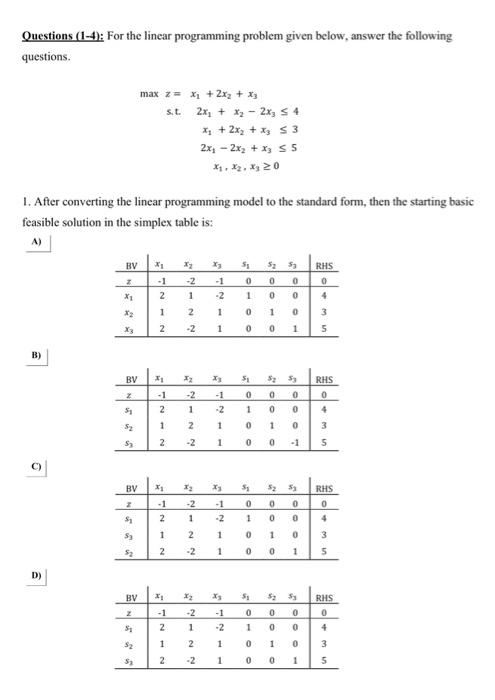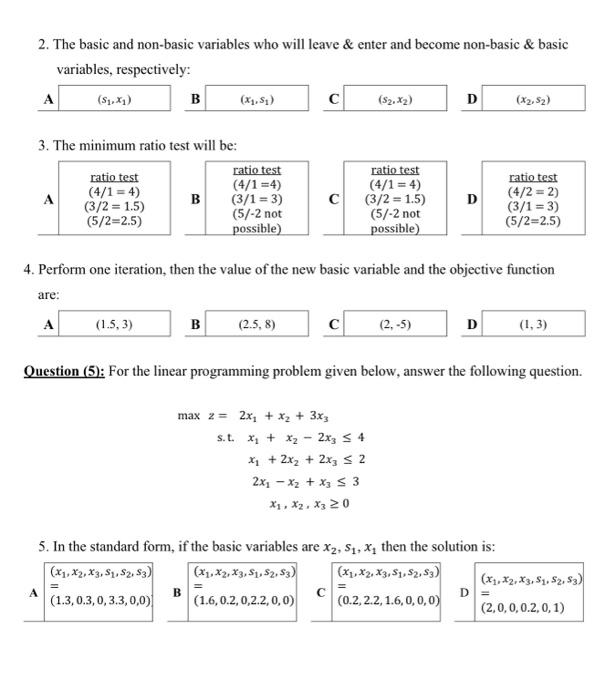# (Solved): Please solve linear programming problems thanks Questions (1-4): For the linear programming problem ...

Please solve linear programming problems thanksQuestions (1-4): For the linear programming problem given below, answer the following questions. $\begin{array}{l} \max z= x_{1}+2 x_{2}+x_{3} \\ \text { s.t. } \quad 2 x_{1}+x_{2}-2 x_{3} \leq 4 \\ x_{1}+2 x_{2}+x_{3} \leq 3 \\ 2 x_{1}-2 x_{2}+x_{3} \leq 5 \\ x_{1}, x_{2}, x_{3} \geq 0 \end{array}$ 1. After converting the linear programming model to the standard form, then the starting basic feasible solution in the simplex table is: 2. The basic and non-basic variables who will leave \& enter and become non-basic \& basic variables, respectively: A ] $$\mathbf{C}$$ $$\mathbf{L}$$ 3. The minimum ratio test will be: \begin{tabular}{|c|c|} \hline \multirow{4}{*}{$$\mathbf{\text { ratio test }}$$} \\ $$(4 / 1=4)$$ \\ $$(3 / 2=1.5)$$ \\ $$(5 / 2=2.5)$$ \\ \hline \end{tabular} B \begin{tabular}{l} ratio test \\ $$(4 / 1=4)$$ \\ $$(3 / 1=3)$$ \\ $$(5 /-2$$ not \\ possible $$)$$ \\ \hline \end{tabular} \begin{tabular}{|c|} \hline ratio test \\ $$(4 / 1=4)$$ \\ $$(3 / 2=1.5)$$ \\ $$(5 /-2$$ not \\ possible) \\ \hline \end{tabular} D \begin{tabular}{l} ratio test \\ $$(4 / 2=2)$$ \\ $$(3 / 1=3)$$ \\ $$(5 / 2=2.5)$$ \\ \hline \end{tabular} 4. Perform one iteration, then the value of the new basic variable and the objective function are: A I C D Question (5): For the linear programming problem given below, answer the following question. $\begin{array}{l} \max z=2 x_{1}+x_{2}+3 x_{3} \\ \text { s.t. } \quad x_{1}+x_{2}-2 x_{3} \leq 4 \\ x_{1}+2 x_{2}+2 x_{3} \leq 2 \\ 2 x_{1}-x_{2}+x_{3} \leq 3 \\ x_{1}, x_{2}, x_{3} \geq 0 \end{array}$ 5. In the standard form, if the basic variables are $$x_{2}, s_{1}, x_{1}$$ then the solution is:

We have an Answer from Expert